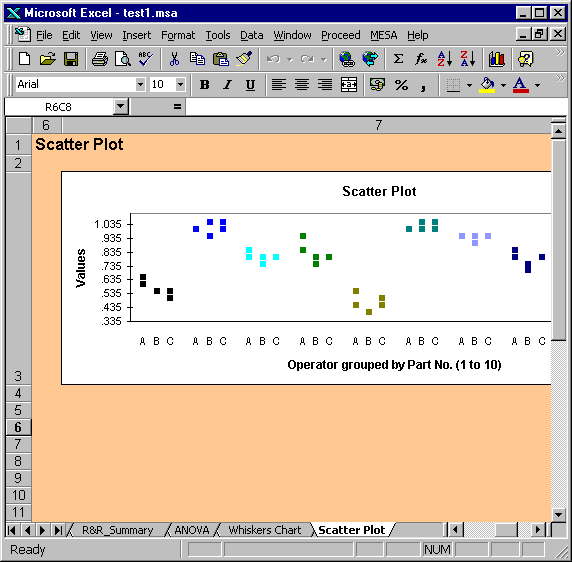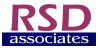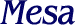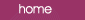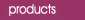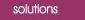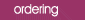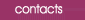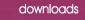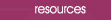Scatter Plot In the Scatter Plot the values are grouped by part number, e.g. all of the readings for part number 1 are plotted together in the first group.  The Scatter Plot for the AIAG data set is shown below. The form of the plot is basically the same as a Normalised Individuals Chart: the main difference being that the actual values are plotted, rather than the normalised values.  The Scatter Plot is useful for assessing: ·       consistency between operators ·       existence of outliers ·       operator-part interactions